GeeksforGeeks App
Open AppBrowser
Continue

# Priority Queue using Binary Heap

Priority Queue is an extension of the queue with the following properties:

1. Every item has a priority associated with it.
2. An element with high priority is dequeued before an element with low priority.
3. If two elements have the same priority, they are served according to their order in the queue.

A Binary Heap is a Binary Tree with the following properties:

1. It is a Complete Tree. This property of Binary Heap makes them suitable to be stored in an array.
2. A Binary Heap is either Min Heap or Max Heap.
3. In a Min Binary Heap, the key at the root must be minimum among all keys present in Binary Heap. The same property must be recursively true for all nodes in Binary Tree.
4. Similarly, in a Max Binary Heap, the key at the root must be maximum among all keys present in Binary Heap. The same property must be recursively true for all nodes in Binary Tree.

### Operation on Binary Heap

• insert(p): Inserts a new element with priority p.
• extractMax(): Extracts an element with maximum priority.
• remove(i): Removes an element pointed by an iterator i.
• getMax(): Returns an element with maximum priority.
• changePriority(i, p): Changes the priority of an element pointed by i to p.

### Example of A Binary Max Heap

• Suppose below is the given Binary Heap that follows all the properties of Binary Max Heap.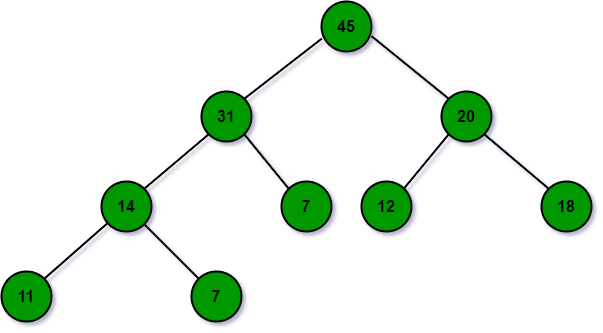• Now a node with value 32 need to be insert in the above heap: To insert an element, attach the new element to any leaf. For Example A node with priority 32 can be added to the leaf of the node 7. But this violates the heap property. To maintain the heap property, shift up the new node 32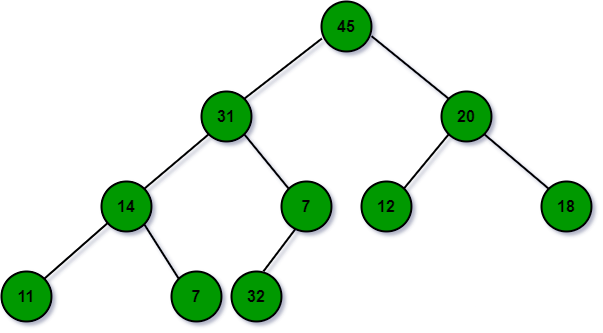• Shift Up Operation get node with 32 at the correct position: Swap the incorrectly placed node with its parent until the heap property is satisfied. For Example: As node 7 is less than node 32 so, swap node 7 and node 32. Then, swap node 31 and node 32.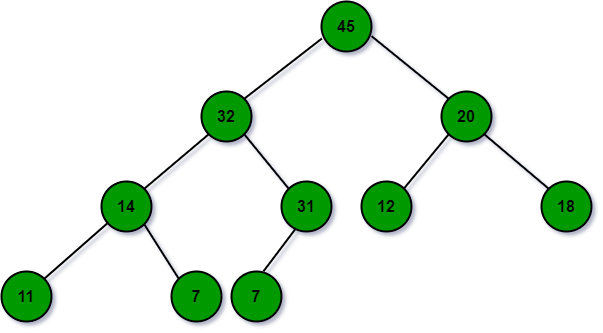• ExtractMax: The maximum value is stored at the root of the tree. But the root of the tree cannot be directly removed. First, it is replaced with any one of the leaves and then removed. For Example: To remove Node 45, it is first replaced with node 7. But this violates the heap property, so move the replaced node down. For that, use shift-down operation.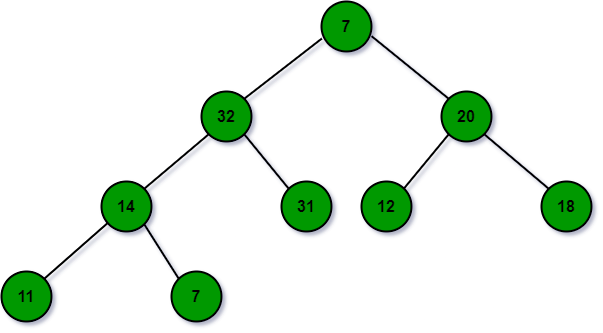• ShiftDown operation: Swap the incorrectly placed node with a larger child until the heap property is satisfied. For Example Node 7 is swapped with node 32 then, last it is swapped with node 31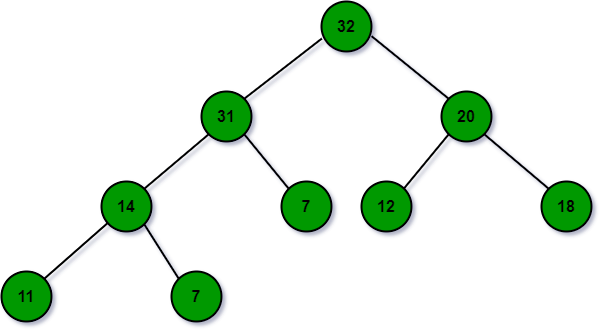• ChangePriority: Let the changed element shift up or down depending on whether its priority decreased or increased. For Example: Change the priority of nodes 11 to 35, due to this change the node has to shift up the node in order to maintain the heap property.
• Remove: To remove an element, change its priority to a value larger than the current maximum, then shift it up, and then extract it using extract max. Find the current maximum using getMax.
• GetMax: The max value is stored at the root of the tree. To getmax, just return the value at the root of the tree.

### Array Representation of Binary Heap

Since the heap is maintained in form of a complete binary tree, because of this fact the heap can be represented in the form of an array. To keep the tree complete and shallow, while inserting a new element insert it in the leftmost vacant position in the last level i.e., at the end of our array. Similarly, while extracting maximum replace the root with the last leaf at the last level i.e., the last element of the array. Below is the illustration of the same: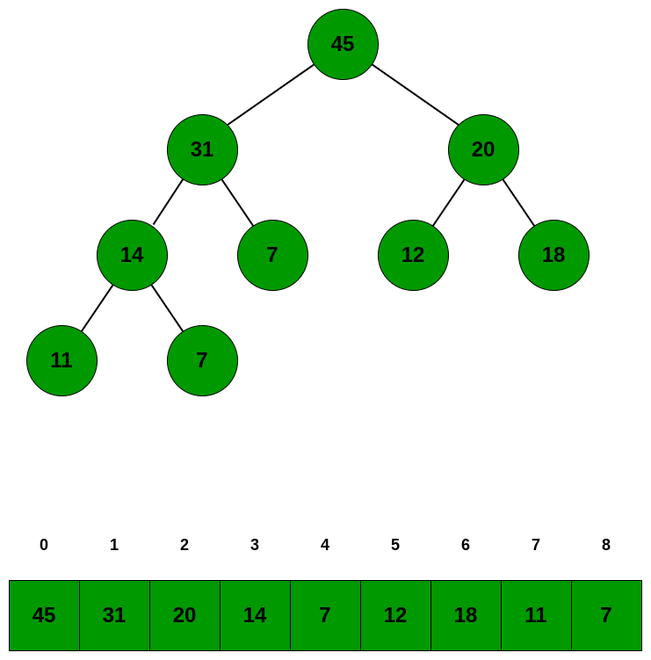Below is the program to implement Priority Queue using Binary Heap:

## C++

 `// C++ code to implement priority-queue``// using array implementation of``// binary heap` `#include ``using` `namespace` `std;` `int` `H;``int` `size = -1;` `// Function to return the index of the``// parent node of a given node``int` `parent(``int` `i)``{` `    ``return` `(i - 1) / 2;``}` `// Function to return the index of the``// left child of the given node``int` `leftChild(``int` `i)``{` `    ``return` `((2 * i) + 1);``}` `// Function to return the index of the``// right child of the given node``int` `rightChild(``int` `i)``{` `    ``return` `((2 * i) + 2);``}` `// Function to shift up the node in order``// to maintain the heap property``void` `shiftUp(``int` `i)``{``    ``while` `(i > 0 && H[parent(i)] < H[i]) {` `        ``// Swap parent and current node``        ``swap(H[parent(i)], H[i]);` `        ``// Update i to parent of i``        ``i = parent(i);``    ``}``}` `// Function to shift down the node in``// order to maintain the heap property``void` `shiftDown(``int` `i)``{``    ``int` `maxIndex = i;` `    ``// Left Child``    ``int` `l = leftChild(i);` `    ``if` `(l <= size && H[l] > H[maxIndex]) {``        ``maxIndex = l;``    ``}` `    ``// Right Child``    ``int` `r = rightChild(i);` `    ``if` `(r <= size && H[r] > H[maxIndex]) {``        ``maxIndex = r;``    ``}` `    ``// If i not same as maxIndex``    ``if` `(i != maxIndex) {``        ``swap(H[i], H[maxIndex]);``        ``shiftDown(maxIndex);``    ``}``}` `// Function to insert a new element``// in the Binary Heap``void` `insert(``int` `p)``{``    ``size = size + 1;``    ``H[size] = p;` `    ``// Shift Up to maintain heap property``    ``shiftUp(size);``}` `// Function to extract the element with``// maximum priority``int` `extractMax()``{``    ``int` `result = H;` `    ``// Replace the value at the root``    ``// with the last leaf``    ``H = H[size];``    ``size = size - 1;` `    ``// Shift down the replaced element``    ``// to maintain the heap property``    ``shiftDown(0);``    ``return` `result;``}` `// Function to change the priority``// of an element``void` `changePriority(``int` `i, ``int` `p)``{``    ``int` `oldp = H[i];``    ``H[i] = p;` `    ``if` `(p > oldp) {``        ``shiftUp(i);``    ``}``    ``else` `{``        ``shiftDown(i);``    ``}``}` `// Function to get value of the current``// maximum element``int` `getMax()``{` `    ``return` `H;``}` `// Function to remove the element``// located at given index``void` `remove``(``int` `i)``{``    ``H[i] = getMax() + 1;` `    ``// Shift the node to the root``    ``// of the heap``    ``shiftUp(i);` `    ``// Extract the node``    ``extractMax();``}` `// Driver Code``int` `main()``{` `    ``/*         45``            ``/      \``           ``31      14``          ``/  \    /  \``         ``13  20  7   11``        ``/  \``       ``12   7``    ``Create a priority queue shown in``    ``example in a binary max heap form.``    ``Queue will be represented in the``    ``form of array as:``    ``45 31 14 13 20 7 11 12 7 */` `    ``// Insert the element to the``    ``// priority queue``    ``insert(45);``    ``insert(20);``    ``insert(14);``    ``insert(12);``    ``insert(31);``    ``insert(7);``    ``insert(11);``    ``insert(13);``    ``insert(7);` `    ``int` `i = 0;` `    ``// Priority queue before extracting max``    ``cout << ``"Priority Queue : "``;``    ``while` `(i <= size) {``        ``cout << H[i] << ``" "``;``        ``i++;``    ``}` `    ``cout << ``"\n"``;` `    ``// Node with maximum priority``    ``cout << ``"Node with maximum priority : "``         ``<< extractMax() << ``"\n"``;` `    ``// Priority queue after extracting max``    ``cout << ``"Priority queue after "``         ``<< ``"extracting maximum : "``;``    ``int` `j = 0;``    ``while` `(j <= size) {``        ``cout << H[j] << ``" "``;``        ``j++;``    ``}` `    ``cout << ``"\n"``;` `    ``// Change the priority of element``    ``// present at index 2 to 49``    ``changePriority(2, 49);``    ``cout << ``"Priority queue after "``         ``<< ``"priority change : "``;``    ``int` `k = 0;``    ``while` `(k <= size) {``        ``cout << H[k] << ``" "``;``        ``k++;``    ``}` `    ``cout << ``"\n"``;` `    ``// Remove element at index 3``    ``remove``(3);``    ``cout << ``"Priority queue after "``         ``<< ``"removing the element : "``;``    ``int` `l = 0;``    ``while` `(l <= size) {``        ``cout << H[l] << ``" "``;``        ``l++;``    ``}``    ``return` `0;``}`

## Java

 `// Java code to implement``// priority-queue using``// array implementation of``// binary heap``import` `java.util.*;``class` `GFG{` `static` `int` `[]H = ``new` `int``[``50``];``static` `int` `size = -``1``;` `// Function to return the index of the``// parent node of a given node``static` `int` `parent(``int` `i)``{``  ``return` `(i - ``1``) / ``2``;``}` `// Function to return the index of the``// left child of the given node``static` `int` `leftChild(``int` `i)``{``  ``return` `((``2` `* i) + ``1``);``}` `// Function to return the index of the``// right child of the given node``static` `int` `rightChild(``int` `i)``{``  ``return` `((``2` `* i) + ``2``);``}` `// Function to shift up the``// node in order to maintain``// the heap property``static` `void` `shiftUp(``int` `i)``{``  ``while` `(i > ``0` `&&``         ``H[parent(i)] < H[i])``  ``{``    ``// Swap parent and current node``    ``swap(parent(i), i);` `    ``// Update i to parent of i``    ``i = parent(i);``  ``}``}` `// Function to shift down the node in``// order to maintain the heap property``static` `void` `shiftDown(``int` `i)``{``  ``int` `maxIndex = i;` `  ``// Left Child``  ``int` `l = leftChild(i);` `  ``if` `(l <= size &&``      ``H[l] > H[maxIndex])``  ``{``    ``maxIndex = l;``  ``}` `  ``// Right Child``  ``int` `r = rightChild(i);` `  ``if` `(r <= size &&``      ``H[r] > H[maxIndex])``  ``{``    ``maxIndex = r;``  ``}` `  ``// If i not same as maxIndex``  ``if` `(i != maxIndex)``  ``{``    ``swap(i, maxIndex);``    ``shiftDown(maxIndex);``  ``}``}` `// Function to insert a``// new element in``// the Binary Heap``static` `void` `insert(``int` `p)``{``  ``size = size + ``1``;``  ``H[size] = p;` `  ``// Shift Up to maintain``  ``// heap property``  ``shiftUp(size);``}` `// Function to extract``// the element with``// maximum priority``static` `int` `extractMax()``{``  ``int` `result = H[``0``];` `  ``// Replace the value``  ``// at the root with``  ``// the last leaf``  ``H[``0``] = H[size];``  ``size = size - ``1``;` `  ``// Shift down the replaced``  ``// element to maintain the``  ``// heap property``  ``shiftDown(``0``);``  ``return` `result;``}` `// Function to change the priority``// of an element``static` `void` `changePriority(``int` `i,``                           ``int` `p)``{``  ``int` `oldp = H[i];``  ``H[i] = p;` `  ``if` `(p > oldp)``  ``{``    ``shiftUp(i);``  ``}``  ``else``  ``{``    ``shiftDown(i);``  ``}``}` `// Function to get value of``// the current maximum element``static` `int` `getMax()``{``  ``return` `H[``0``];``}` `// Function to remove the element``// located at given index``static` `void` `remove(``int` `i)``{``  ``H[i] = getMax() + ``1``;` `  ``// Shift the node to the root``  ``// of the heap``  ``shiftUp(i);` `  ``// Extract the node``  ``extractMax();``}``  ` `static` `void` `swap(``int` `i, ``int` `j)``{``  ``int` `temp= H[i];``  ``H[i] = H[j];``  ``H[j] = temp;``}` `// Driver Code``public` `static` `void` `main(String[] args)``{` `  ``/*           45``            ``/        \``           ``31      14``          ``/  \    /  \``         ``13  20  7   11``        ``/  \``       ``12   7``    ``Create a priority queue shown in``    ``example in a binary max heap form.``    ``Queue will be represented in the``    ``form of array as:``    ``45 31 14 13 20 7 11 12 7 */` `  ``// Insert the element to the``  ``// priority queue``  ``insert(``45``);``  ``insert(``20``);``  ``insert(``14``);``  ``insert(``12``);``  ``insert(``31``);``  ``insert(``7``);``  ``insert(``11``);``  ``insert(``13``);``  ``insert(``7``);` `  ``int` `i = ``0``;` `  ``// Priority queue before extracting max``  ``System.out.print(``"Priority Queue : "``);``  ``while` `(i <= size)``  ``{``    ``System.out.print(H[i] + ``" "``);``    ``i++;``  ``}` `  ``System.out.print(``"\n"``);` `  ``// Node with maximum priority``  ``System.out.print(``"Node with maximum priority : "` `+``                    ``extractMax() + ``"\n"``);` `  ``// Priority queue after extracting max``  ``System.out.print(``"Priority queue after "` `+``                   ``"extracting maximum : "``);``  ``int` `j = ``0``;``  ``while` `(j <= size)``  ``{``    ``System.out.print(H[j] + ``" "``);``    ``j++;``  ``}` `  ``System.out.print(``"\n"``);` `  ``// Change the priority of element``  ``// present at index 2 to 49``  ``changePriority(``2``, ``49``);``  ``System.out.print(``"Priority queue after "` `+``                   ``"priority change : "``);``  ``int` `k = ``0``;``  ``while` `(k <= size)``  ``{``    ``System.out.print(H[k] + ``" "``);``    ``k++;``  ``}` `  ``System.out.print(``"\n"``);` `  ``// Remove element at index 3``  ``remove(``3``);``  ``System.out.print(``"Priority queue after "` `+``                   ``"removing the element : "``);``  ``int` `l = ``0``;``  ``while` `(l <= size)``  ``{``    ``System.out.print(H[l] + ``" "``);``    ``l++;``  ``}``}``}` `// This code is contributed by 29AjayKumar`

## Python3

 `# Python3 code to implement priority-queue``# using array implementation of``# binary heap` `H ``=` `[``0``]``*``50``size ``=` `-``1``  ` `# Function to return the index of the``# parent node of a given node``def` `parent(i) :` `    ``return` `(i ``-` `1``) ``/``/` `2``  ` `# Function to return the index of the``# left child of the given node``def` `leftChild(i) :` `    ``return` `((``2` `*` `i) ``+` `1``)``  ` `# Function to return the index of the``# right child of the given node``def` `rightChild(i) :` `    ``return` `((``2` `*` `i) ``+` `2``)``    ` `# Function to shift up the ``# node in order to maintain ``# the heap property``def` `shiftUp(i) :` `    ``while` `(i > ``0` `and` `H[parent(i)] < H[i]) :``          ` `        ``# Swap parent and current node``        ``swap(parent(i), i)``      ` `        ``# Update i to parent of i``        ``i ``=` `parent(i)``        ` `# Function to shift down the node in``# order to maintain the heap property``def` `shiftDown(i) :` `    ``maxIndex ``=` `i``      ` `    ``# Left Child``    ``l ``=` `leftChild(i)``      ` `    ``if` `(l <``=` `size ``and` `H[l] > H[maxIndex]) :``    ` `        ``maxIndex ``=` `l``      ` `    ``# Right Child``    ``r ``=` `rightChild(i)``      ` `    ``if` `(r <``=` `size ``and` `H[r] > H[maxIndex]) :``    ` `        ``maxIndex ``=` `r``      ` `    ``# If i not same as maxIndex``    ``if` `(i !``=` `maxIndex) :``    ` `        ``swap(i, maxIndex)``        ``shiftDown(maxIndex)``        ` `# Function to insert a ``# new element in ``# the Binary Heap``def` `insert(p) :``    ` `    ``global` `size``    ``size ``=` `size ``+` `1``    ``H[size] ``=` `p``      ` `    ``# Shift Up to maintain ``    ``# heap property``    ``shiftUp(size)``  ` `# Function to extract ``# the element with``# maximum priority``def` `extractMax() :``    ` `    ``global` `size``    ``result ``=` `H[``0``]``      ` `    ``# Replace the value ``    ``# at the root with ``    ``# the last leaf``    ``H[``0``] ``=` `H[size]``    ``size ``=` `size ``-` `1``      ` `    ``# Shift down the replaced ``    ``# element to maintain the ``    ``# heap property``    ``shiftDown(``0``)``    ``return` `result``  ` `# Function to change the priority``# of an element``def` `changePriority(i,p) :` `    ``oldp ``=` `H[i]``    ``H[i] ``=` `p``      ` `    ``if` `(p > oldp) :``    ` `        ``shiftUp(i)`` ` `    ``else` `:``    ` `        ``shiftDown(i)``  ` `# Function to get value of ``# the current maximum element``def` `getMax() :`` ` `    ``return` `H[``0``]``  ` `# Function to remove the element``# located at given index``def` `Remove(i) :` `    ``H[i] ``=` `getMax() ``+` `1``      ` `    ``# Shift the node to the root``    ``# of the heap``    ``shiftUp(i)``      ` `    ``# Extract the node``    ``extractMax()``  ` `def` `swap(i, j) :``    ` `    ``temp ``=` `H[i]``    ``H[i] ``=` `H[j]``    ``H[j] ``=` `temp``    ` `# Insert the element to the``# priority queue``insert(``45``)``insert(``20``)``insert(``14``)``insert(``12``)``insert(``31``)``insert(``7``)``insert(``11``)``insert(``13``)``insert(``7``)``  ` `i ``=` `0``  ` `# Priority queue before extracting max``print``(``"Priority Queue : "``, end ``=` `"")``while` `(i <``=` `size) :` `    ``print``(H[i], end ``=` `" "``)``    ``i ``+``=` `1``  ` `print``()``  ` `# Node with maximum priority``print``(``"Node with maximum priority :"` `,  extractMax())``  ` `# Priority queue after extracting max``print``(``"Priority queue after extracting maximum : "``, end ``=` `"")``j ``=` `0``while` `(j <``=` `size) :` `    ``print``(H[j], end ``=` `" "``)``    ``j ``+``=` `1``  ` `print``()``  ` `# Change the priority of element``# present at index 2 to 49``changePriority(``2``, ``49``)``print``(``"Priority queue after priority change : "``, end ``=` `"")``k ``=` `0``while` `(k <``=` `size) :` `    ``print``(H[k], end ``=` `" "``)``    ``k ``+``=` `1``  ` `print``()``  ` `# Remove element at index 3``Remove(``3``)``print``(``"Priority queue after removing the element : "``, end ``=` `"")``l ``=` `0``while` `(l <``=` `size) :` `    ``print``(H[l], end ``=` `" "``)``    ``l ``+``=` `1``    ` `    ``# This code is contributed by divyeshrabadiya07.`

## C#

 `// C# code to implement priority-queue``// using array implementation of``// binary heap``using` `System;` `class` `GFG{` `static` `int` `[]H = ``new` `int``;``static` `int` `size = -1;` `// Function to return the index of the``// parent node of a given node``static` `int` `parent(``int` `i)``{``    ``return` `(i - 1) / 2;``}` `// Function to return the index of the``// left child of the given node``static` `int` `leftChild(``int` `i)``{``    ``return` `((2 * i) + 1);``}` `// Function to return the index of the``// right child of the given node``static` `int` `rightChild(``int` `i)``{``    ``return` `((2 * i) + 2);``}` `// Function to shift up the``// node in order to maintain``// the heap property``static` `void` `shiftUp(``int` `i)``{``    ``while` `(i > 0 &&``           ``H[parent(i)] < H[i])``    ``{``        ` `        ``// Swap parent and current node``        ``swap(parent(i), i);``    ` `        ``// Update i to parent of i``        ``i = parent(i);``    ``}``}` `// Function to shift down the node in``// order to maintain the heap property``static` `void` `shiftDown(``int` `i)``{``    ``int` `maxIndex = i;``    ` `    ``// Left Child``    ``int` `l = leftChild(i);``    ` `    ``if` `(l <= size &&``        ``H[l] > H[maxIndex])``    ``{``        ``maxIndex = l;``    ``}``    ` `    ``// Right Child``    ``int` `r = rightChild(i);``    ` `    ``if` `(r <= size &&``        ``H[r] > H[maxIndex])``    ``{``        ``maxIndex = r;``    ``}``    ` `    ``// If i not same as maxIndex``    ``if` `(i != maxIndex)``    ``{``        ``swap(i, maxIndex);``        ``shiftDown(maxIndex);``    ``}``}` `// Function to insert a``// new element in``// the Binary Heap``static` `void` `insert(``int` `p)``{``    ``size = size + 1;``    ``H[size] = p;``    ` `    ``// Shift Up to maintain``    ``// heap property``    ``shiftUp(size);``}` `// Function to extract``// the element with``// maximum priority``static` `int` `extractMax()``{``    ``int` `result = H;``    ` `    ``// Replace the value``    ``// at the root with``    ``// the last leaf``    ``H = H[size];``    ``size = size - 1;``    ` `    ``// Shift down the replaced``    ``// element to maintain the``    ``// heap property``    ``shiftDown(0);``    ``return` `result;``}` `// Function to change the priority``// of an element``static` `void` `changePriority(``int` `i,``                           ``int` `p)``{``    ``int` `oldp = H[i];``    ``H[i] = p;``    ` `    ``if` `(p > oldp)``    ``{``        ``shiftUp(i);``    ``}``    ``else``    ``{``        ``shiftDown(i);``    ``}``}` `// Function to get value of``// the current maximum element``static` `int` `getMax()``{``    ``return` `H;``}` `// Function to remove the element``// located at given index``static` `void` `Remove(``int` `i)``{``    ``H[i] = getMax() + 1;``    ` `    ``// Shift the node to the root``    ``// of the heap``    ``shiftUp(i);``    ` `    ``// Extract the node``    ``extractMax();``}` `static` `void` `swap(``int` `i, ``int` `j)``{``    ``int` `temp = H[i];``    ``H[i] = H[j];``    ``H[j] = temp;``}` `// Driver Code``public` `static` `void` `Main(String[] args)``{` `/*              45``            ``/     \``           ``31      14``          ``/ \     / \``        ``13  20   7   11``       ``/ \``      ``12  7``    ``Create a priority queue shown in``    ``example in a binary max heap form.``    ``Queue will be represented in the``    ``form of array as:``    ``45 31 14 13 20 7 11 12 7 */` `    ``// Insert the element to the``    ``// priority queue``    ``insert(45);``    ``insert(20);``    ``insert(14);``    ``insert(12);``    ``insert(31);``    ``insert(7);``    ``insert(11);``    ``insert(13);``    ``insert(7);``    ` `    ``int` `i = 0;``    ` `    ``// Priority queue before extracting max``    ``Console.Write(``"Priority Queue : "``);``    ``while` `(i <= size)``    ``{``        ``Console.Write(H[i] + ``" "``);``        ``i++;``    ``}``    ` `    ``Console.Write(``"\n"``);``    ` `    ``// Node with maximum priority``    ``Console.Write(``"Node with maximum priority : "` `+``                   ``extractMax() + ``"\n"``);``    ` `    ``// Priority queue after extracting max``    ``Console.Write(``"Priority queue after "` `+``                  ``"extracting maximum : "``);``    ``int` `j = 0;``    ``while` `(j <= size)``    ``{``        ``Console.Write(H[j] + ``" "``);``        ``j++;``    ``}``    ` `    ``Console.Write(``"\n"``);``    ` `    ``// Change the priority of element``    ``// present at index 2 to 49``    ``changePriority(2, 49);``    ``Console.Write(``"Priority queue after "` `+``                  ``"priority change : "``);``    ``int` `k = 0;``    ``while` `(k <= size)``    ``{``        ``Console.Write(H[k] + ``" "``);``        ``k++;``    ``}``    ` `    ``Console.Write(``"\n"``);``    ` `    ``// Remove element at index 3``    ``Remove(3);``    ``Console.Write(``"Priority queue after "` `+``                  ``"removing the element : "``);``    ``int` `l = 0;``    ``while` `(l <= size)``    ``{``        ``Console.Write(H[l] + ``" "``);``        ``l++;``    ``}``}``}` `// This code is contributed by Amit Katiyar`

## Javascript

 ``

Output

```Priority Queue : 45 31 14 13 20 7 11 12 7
Node with maximum priority : 45
Priority queue after extracting maximum : 31 20 14 13 7 7 11 12
Priority queue after priority change : 49 20 31 13 7 7 11 12
Priority queue after removing the element : 49 20 31 12 7 7 11 ```

Time Complexity: The time complexity of all the operation is O(log N) except for GetMax() which has time complexity of O(1).
Auxiliary Space: O(N)

Method – 2

below is also a valid method to implement this priority queue using a max heap. this code is a generic method to implement a priority queue using a class-based structure. here in the implementation part, we are using a generic template (not a specific data type) so this implementation works for all data types.

## C++

 `#include ``#include ` `using` `namespace` `std;` `// Priority queue implementation in C++``template``<``typename` `T>``class` `PriorityQueue {``  ``private``:``  ``vector data;` `  ``public``:``  ``// Implementing Priority Queue using inbuilt available vector in C++``  ``PriorityQueue() {}` `  ``// Element Inserting function``  ``void` `Enqueue(T item) {``    ``// item Insertion``    ``data.push_back(item);``    ``int` `ci = data.size() - 1;` `    ``// Re-structure heap(Max Heap) so that after``    ``// addition max element will lie on top of pq``    ``while` `(ci > 0) {``      ``int` `pi = (ci - 1) / 2;``      ``if` `(data[ci] <= data[pi])``        ``break``;``      ``T tmp = data[ci];``      ``data[ci] = data[pi];``      ``data[pi] = tmp;``      ``ci = pi;``    ``}``  ``}` `  ``T Dequeue() {``    ``// deleting top element of pq``    ``int` `li = data.size() - 1;``    ``T frontItem = data;``    ``data = data[li];``    ``data.pop_back();` `    ``--li;``    ``int` `pi = 0;` `    ``// Re-structure heap(Max Heap) so that after``    ``// deletion max element will lie on top of pq``    ``while` `(``true``) {``      ``int` `ci = pi * 2 + 1;``      ``if` `(ci > li)``        ``break``;``      ``int` `rc = ci + 1;``      ``if` `(rc <= li && data[rc] < data[ci])``        ``ci = rc;``      ``if` `(data[pi] >= data[ci])``        ``break``;``      ``T tmp = data[pi];``      ``data[pi] = data[ci];``      ``data[ci] = tmp;``      ``pi = ci;``    ``}``    ``return` `frontItem;``  ``}` `  ``// Function which returns peek element``  ``T Peek() {``    ``T frontItem = data;``    ``return` `frontItem;``  ``}` `  ``int` `Count() {``    ``return` `data.size();``  ``}``};` `// Driver code``int` `main()``{``  ` `  ``// Basic functionality sample of Priority Queue` `  ``// Creating priority queue which contains int in it``  ``PriorityQueue<``int``> pq;` `  ``// Insert element 1 in pq``  ``pq.Enqueue(1);` `  ``cout << ``"Size of pq is : "` `<< pq.Count() <<``    ``" and Peek Element is : "` `<< pq.Peek() << endl;` `  ``// Insert element 10 and -8 in pq``  ``pq.Enqueue(10);``  ``pq.Enqueue(-8);` `  ``cout << ``"Size of pq is : "` `<< pq.Count() <<``    ``" and Peek Element is : "` `<< pq.Peek() << endl;` `  ``// Delete element from pq``  ``pq.Dequeue();` `  ``cout << ``"Size of pq is : "` `<< pq.Count() <<``    ``" and Peek Element is : "` `<< pq.Peek() << endl;` `  ``// Delete element from pq``  ``pq.Dequeue();` `  ``cout << ``"Size of pq is : "` `<< pq.Count() <<``    ``" and Peek Element is : "` `<< pq.Peek() << endl;` `  ``// Insert element 25 in pq``  ``pq.Enqueue(25);` `  ``cout << ``"Size of pq is : "` `<< pq.Count() <<``    ``" and Peek Element is : "` `<< pq.Peek() << endl;` `  ``return` `0;``}`

## Java

 `import` `java.util.ArrayList;``import` `java.util.List;` `public` `class` `GFG {``  ` `// Priority queue implementation in Java``static` `class` `PriorityQueue> {``    ``private` `List data;` `    ``// Implementing Priority Queue using inbuilt available List in Java``    ``public` `PriorityQueue() {``        ``this``.data = ``new` `ArrayList();``    ``}` `    ``// Element Inserting function``    ``public` `void` `Enqueue(T item) {``        ``// item Insertion``        ``data.add(item);``        ``int` `ci = data.size() - ``1``;` `        ``// Re-structure heap(Max Heap) so that after addition max element will lie on top of pq``        ``while` `(ci > ``0``) {``            ``int` `pi = (ci - ``1``) / ``2``;``            ``if` `(data.get(ci).compareTo(data.get(pi)) <= ``0``)``                ``break``;``            ``T tmp = data.get(ci);``            ``data.set(ci, data.get(pi));``            ``data.set(pi, tmp);``            ``ci = pi;``        ``}``    ``}` `    ``public` `T Dequeue() {``        ``// deleting top element of pq``        ``int` `li = data.size() - ``1``;``        ``T frontItem = data.get(``0``);``        ``data.set(``0``, data.get(li));``        ``data.remove(li);` `        ``--li;``        ``int` `pi = ``0``;` `        ``// Re-structure heap(Max Heap) so that after deletion max element will lie on top of pq``        ``while` `(``true``) {``            ``int` `ci = pi * ``2` `+ ``1``;``            ``if` `(ci > li)``                ``break``;``            ``int` `rc = ci + ``1``;``            ``if` `(rc <= li && data.get(rc).compareTo(data.get(ci)) < ``0``)``                ``ci = rc;``            ``if` `(data.get(pi).compareTo(data.get(ci)) >= ``0``)``                ``break``;``            ``T tmp = data.get(pi);``            ``data.set(pi, data.get(ci));``            ``data.set(ci, tmp);``            ``pi = ci;``        ``}``        ``return` `frontItem;``    ``}` `    ``// Function which returns peek element``    ``public` `T Peek() {``        ``T frontItem = data.get(``0``);``        ``return` `frontItem;``    ``}` `    ``public` `int` `Count() {``        ``return` `data.size();``    ``}``}` `// Driver code``public` `static` `void` `main(String[] args) {``    ``// Basic functionality sample of Priority Queue` `    ``// Creating priority queue which contains int in it``    ``PriorityQueue pq = ``new` `PriorityQueue();` `    ``// Insert element 1 in pq``    ``pq.Enqueue(``1``);` `    ``System.out.println(``"Size of pq is : "` `+ pq.Count() + ``" and Peek Element is : "` `+ pq.Peek());` `    ``// Insert element 10 and -8 in pq``    ``pq.Enqueue(``10``);``    ``pq.Enqueue(-``8``);` `    ``System.out.println(``"Size of pq is : "` `+ pq.Count() + ``" and Peek Element is : "` `+ pq.Peek());` `    ``// Delete element from pq``    ``pq.Dequeue();` `    ``System.out.println(``"Size of pq is : "` `+ pq.Count() + ``" and Peek Element is : "` `+ pq.Peek());` `    ``// Delete element from pq``    ``pq.Dequeue();` `    ``System.out.println(``"Size of pq is : "` `+ pq.Count() + ``" and Peek Element is : "` `+ pq.Peek());` `    ``// Insert element 25 in pq``    ``pq.Enqueue(``25``);` `    ``System.out.println(``"Size of pq is : "` `+ pq.Count() + ``" and Peek Element is : "` `+ pq.Peek());``}``}`

## Python3

 `import` `heapq` `class` `PriorityQueue:``    ``def` `__init__(``self``):``        ``self``._queue ``=` `[]``        ``self``._index ``=` `0``    ` `    ``def` `enqueue(``self``, item, priority):``        ``heapq.heappush(``self``._queue, (priority, ``self``._index, item))``        ``self``._index ``+``=` `1``    ` `    ``def` `dequeue(``self``):``        ``return` `heapq.heappop(``self``._queue)[``-``1``]``    ` `    ``def` `peek(``self``):``        ``return` `self``._queue[``0``][``-``1``]``    ` `    ``def` `count(``self``):``        ``return` `len``(``self``._queue)``        ` `# Driver code``if` `__name__ ``=``=` `"__main__"``:``    ``# Basic functionality sample of Priority Queue` `    ``# Creating priority queue which contains int in it``    ``pq ``=` `PriorityQueue()` `    ``# Insert element 1 in pq``    ``pq.enqueue(``1``, ``1``)` `    ``print``(``"Size of pq is : "``, pq.count(), ``" and Peek Element is : "``, pq.peek())` `    ``# Insert element 10 and -8 in pq``    ``pq.enqueue(``10``, ``2``)``    ``pq.enqueue(``-``8``, ``3``)` `    ``print``(``"Size of pq is : "``, pq.count(), ``" and Peek Element is : "``, pq.peek())` `    ``# Delete element from pq``    ``pq.dequeue()` `    ``print``(``"Size of pq is : "``, pq.count(), ``" and Peek Element is : "``, pq.peek())` `    ``# Delete element from pq``    ``pq.dequeue()` `    ``print``(``"Size of pq is : "``, pq.count(), ``" and Peek Element is : "``, pq.peek())` `    ``# Insert element 25 in pq``    ``pq.enqueue(``25``, ``4``)` `    ``print``(``"Size of pq is : "``, pq.count(), ``" and Peek Element is : "``, pq.peek())`

## C#

 `using` `System;``using` `System.Collections.Generic;` `class` `GFG {` `    ``// Priority queue implementation in C#``    ``class` `PriorityQueue ``where` `T : IComparable {``        ``private` `List data;` `        ``// Implemeting Priority Queue using inbuilt available List in C#``        ``public` `PriorityQueue() {``            ``this``.data = ``new` `List();``        ``}` `        ``// Element Inserting function``        ``public` `void` `Enqueue(T item) {``            ``// item Insertion``            ``data.Add(item);``            ``int` `ci = data.Count - 1;` `            ``// re-structure heap(Max Heap) so that after addition max element will lie on top of pq``            ``while` `(ci > 0) {``                ``int` `pi = (ci - 1) / 2;``                ``if` `(data[ci].CompareTo(data[pi]) <= 0)``                    ``break``;``                ``T tmp = data[ci]; data[ci] = data[pi]; data[pi] = tmp;``                ``ci = pi;``            ``}``        ``}` `        ``public` `T Dequeue() {``            ``// deleting top element of pq``            ``int` `li = data.Count - 1;``            ``T frontItem = data;``            ``data = data[li];``            ``data.RemoveAt(li);` `            ``--li;``            ``int` `pi = 0;` `            ``// re-structure heap(Max Heap) so that after deletion max element will lie on top of pq``            ``while` `(``true``) {``                ``int` `ci = pi * 2 + 1;``                ``if` `(ci > li) ``break``;``                ``int` `rc = ci + 1;``                ``if` `(rc <= li && data[rc].CompareTo(data[ci]) < 0)``                    ``ci = rc;``                ``if` `(data[pi].CompareTo(data[ci]) >= 0) ``break``;``                ``T tmp = data[pi]; data[pi] = data[ci]; data[ci] = tmp;``                ``pi = ci;``            ``}``            ``return` `frontItem;``        ``}` `        ``// function which returns peek element``        ``public` `T Peek() {``            ``T frontItem = data;``            ``return` `frontItem;``        ``}` `        ``public` `int` `Count() {``            ``return` `data.Count;``        ``}``    ``}` `    ``// Driver code``    ``public` `static` `void` `Main(``string``[] args) {``        ``// basic functionality sample of Priority Queue` `        ``// creating priority queue which contains int in it``        ``var` `pq = ``new` `PriorityQueue<``int``>();` `        ``// Insert element 1 in pq``        ``pq.Enqueue(1);` `        ``Console.WriteLine(``"Size of pq is : "` `+ pq.Count() + ``" and Peek Element is : "` `+ pq.Peek());` `        ``// Insert element 10 and -8 in pq``        ``pq.Enqueue(10);``        ``pq.Enqueue(-8);` `        ``Console.WriteLine(``"Size of pq is : "` `+ pq.Count() + ``" and Peek Element is : "` `+ pq.Peek());` `        ``// Delete element from pq``        ``pq.Dequeue();` `        ``Console.WriteLine(``"Size of pq is : "` `+ pq.Count() + ``" and Peek Element is : "` `+ pq.Peek());` `        ``// Delete element from pq``        ``pq.Dequeue();` `        ``Console.WriteLine(``"Size of pq is : "` `+ pq.Count() + ``" and Peek Element is : "` `+ pq.Peek());` `        ``// Insert element 25 in pq``        ``pq.Enqueue(25);` `        ``Console.WriteLine(``"Size of pq is : "` `+ pq.Count() + ``" and Peek Element is : "` `+ pq.Peek());``    ``}``}`

## Javascript

 `// Priority queue implementation in JavaScript``class PriorityQueue {``  ``constructor() {``    ``this``.data = [];``  ``}` `  ``// Element Inserting function``  ``enqueue(item) {``    ``// item Insertion``    ``this``.data.push(item);``    ``let ci = ``this``.data.length - 1;` `    ``// Re-structure heap(Max Heap) so that after``    ``// addition max element will lie on top of pq``    ``while` `(ci > 0) {``      ``let pi = Math.floor((ci - 1) / 2);``      ``if` `(``this``.data[ci] <= ``this``.data[pi])``        ``break``;``      ``let tmp = ``this``.data[ci];``      ``this``.data[ci] = ``this``.data[pi];``      ``this``.data[pi] = tmp;``      ``ci = pi;``    ``}``  ``}` `  ``dequeue() {``    ``// deleting top element of pq``    ``let li = ``this``.data.length - 1;``    ``let frontItem = ``this``.data;``    ``this``.data = ``this``.data[li];``    ``this``.data.pop();` `    ``--li;``    ``let pi = 0;` `    ``// Re-structure heap(Max Heap) so that after``    ``// deletion max element will lie on top of pq``    ``while` `(``true``) {``      ``let ci = pi * 2 + 1;``      ``if` `(ci > li)``        ``break``;``      ``let rc = ci + 1;``      ``if` `(rc <= li && ``this``.data[rc] < ``this``.data[ci])``        ``ci = rc;``      ``if` `(``this``.data[pi] >= ``this``.data[ci])``        ``break``;``      ``let tmp = ``this``.data[pi];``      ``this``.data[pi] = ``this``.data[ci];``      ``this``.data[ci] = tmp;``      ``pi = ci;``    ``}``    ``return` `frontItem;``  ``}` `  ``// Function which returns peek element``  ``peek() {``    ``let frontItem = ``this``.data;``    ``return` `frontItem;``  ``}` `  ``count() {``    ``return` `this``.data.length;``  ``}``}` `// Driver code``let pq = ``new` `PriorityQueue();` `// Basic functionality sample of Priority Queue` `// Insert element 1 in pq``pq.enqueue(1);` `console.log(`Size of pq is: \${pq.count()} and Peek Element is: \${pq.peek()}`);` `// Insert element 10 and -8 in pq``pq.enqueue(10);``pq.enqueue(-8);` `console.log(`Size of pq is: \${pq.count()} and Peek Element is: \${pq.peek()}`);` `// Delete element from pq``pq.dequeue();` `console.log(`Size of pq is: \${pq.count()} and Peek Element is: \${pq.peek()}`);` `// Delete element from pq``pq.dequeue();` `console.log(`Size of pq is: \${pq.count()} and Peek Element is: \${pq.peek()}`);` `// Insert element 25 in pq``pq.enqueue(25);` `console.log(`Size of pq is: \${pq.count()} and Peek Element is: \${pq.peek()}`);`

Output

```Size of pq is : 1 and Peek Element is : 1
Size of pq is : 3 and Peek Element is : 10
Size of pq is : 2 and Peek Element is : 1
Size of pq is : 1 and Peek Element is : -8
Size of pq is : 2 and Peek Element is : 25```

Time Complexity: O(log(n)) for EnQueue operation and O(log(n)) for Dequeue operation
Space complexity: O(n) (as we need n size array for implementation)

My Personal Notes arrow_drop_up